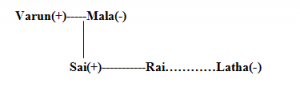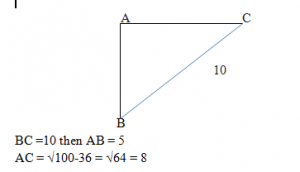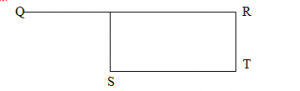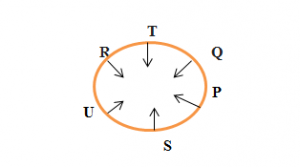#Reasoning Questions: Data Sufficiency Set 16

Hello Aspirants,

Welcome to Online Quantitative Aptitude section in AffairsCloud.com. Here we are creating question sample From DATA SUFFICIENCY topic, which is common for IBPS, SBI, RBI, IPPB, LIC , SSC and  other competitive exams. We have included Some questions that are repeatedly asked in exams.

1. Who among A, B, C, D and E is the highest ?
I.B is heavier than A and D, and heavier than E
II.A is heavier than B and lighter than D
III.C is lighter than E
1.Only I
2.Both I and  II
3.Both I and III
4.Either I or III
5.All of these
Answer – 3.Both I and III
Explanation :
From I:
E<B<(A,D)
From III
C<E

2. How many children are there in the row of  facing North ?
I.Rani who is eighth from the left end is sixth to the left of Raja who is fourteenth from the right end
II.Rahul is fifth to the left of Neeli who is tenth from the right end and fifteenth from the left end
1.Only I
2.Only II
3.Both I and II
4.Either I or II
5.Neither I or II
Answer – 4.Either I or II
Explanation :
From I :
Total  = 8+20-1 = 24
From II :
10+15-1 =24

3. A, M, W, R and I are sitting in a row. If R is sitting between I and A, who is sitting in the middle(All are facing North ) ?
I.W always sitting at either of the extreme ends. Neither R nor M is an immediate neighbour of W
II.I is sitting to the right of M, but on the left of R
1.Only I
2.Only II
3.Both I and II
4.Either I or II
5.Neither I or II
Explanation :
From I Two possibility rises
WIRAM
MIRAW

4. How is Rai related to Varun ?
I.Sai is one of the brothers of Rai. Latha is the sister of Rai
II.Varun is husband of Mala, who is mother of Sai
1.Only I
2.Only II
3.Both I and II
4.Either I or II
5.Neither I or II
Answer – 5.Neither I or II
Explanation :
Rai is either daughter or son of Varun.5. How many students did secure First class in a class of 50 students ?
I.Double the number of first class students secured second class and all the remaining students failed
II.Number of students failed was twice the number of first class students in the class
1.Only I
2.Only II
3.Both I and II
4.Either I or II
5.Neither I or II
Answer – 3.Both I and II
Explanation :
From I:
No of Students Failed = 50-3x
From II:
No of Students Failed = 2x
2x = 50-3x
5x=50
X = 10

6. If point A is directly north of point B and directly west of point C, what is the distance from point A to point C?
I.The distance from B to C is 10 cm.
II.The distance from A to B is 4 cm less than the distance from B to C.
1.Only I
2.Only II
3.Both I and II
4.Either I or II
5.Neither I or II
Explanation :7. In which direction point T with respect to point Q ?
I.Point S is 7 to m to the South-west of point Q and point S and T form a straight line
II.Point Q and R form a straight line and point R is north to the point of T
1.Only I
2.Only II
3.Both I and II
4.Either I or II
5.Neither I or II
Answer – 5.Neither I or II
Explanation :Exact direction we cant find from the given data

8. In which year was Sugan born ?
I.Sugan’s present age is 20 years more than his child
II.Sugan’s have two children. First child was born in 1993 ?
1.Only I
2.Only II
3.Both I and II
4.Either I or II
5.Neither I or II
Answer – 5.Neither I or II
Explanation :
From I:
S = 20+Which chid(not metioned)
From II:

9. What is the rank of Anil from the bottom in a class of 30 students ?
I.Kani is sixth from the top and there are five students between Kani and Anil.
II.The rank of Jit is fourth from the top and there are 10 students between Jit and Anil.
1.Only I
2.Only II
3.Both I and II
4.Either I or II
5.Neither I or II
Answer – 4.Either I or II
Explanation :
From I
Kani(25)____________Anil(19)
From II
Top.Jit(4)……………………….15(Anil) so rank 16th from the bottom

10. Among P, Q, R, S, T and U who is second o the left of Q. All are sitting around a circular table facing Centre ?
I.S sits opposite  to T and is the immediate neighbours of U an P. R is third to the left of P
II.T is immediate neighbours of Q and R. U is second to the left of P
1.Only I
2.Only II
3.Both I and II
4.Either I or II
5.Neither I or II
Answer – 3.Both I and II
Explanation :